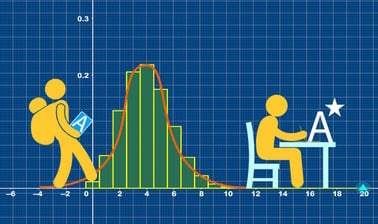# Imperial College London: A-level Mathematics for Year 12 - Course 2: Calculus, Newton’s Laws and Hypothesis Testing

Develop your thinking skills, fluency and confidence to aim for an A* in A-level mathematics and prepare for undergraduate STEM degrees.7 weeks
2–4 hours per week
Self-paced
Free

This course by Imperial College London is designed to help you develop the skills you need to succeed in your A-level mathematics exams.You’ll also be encouraged to consider how what you know fits into the wider mathematical world.

Over seven modules, covering an introduction to calculus, Newton’s laws and statistical hypothesis testing your initial skillset will be extended to give a clear understanding of how background knowledge underpins the A -level course.

You will investigate key topic areas to gain a deeper understanding of the skills and techniques that you can apply throughout your A-level study. These skills include:

• Fluency – selecting and applying correct methods to answer with speed and efficiency
• Confidence – critically assessing mathematical methods and investigating ways to apply them
• Problem solving – analysing the ‘unfamiliar’ and identifying which skills and techniques you require to answer questions
• Constructing mathematical argument – using mathematical tools such as diagrams, graphs, logical deduction, mathematical symbols, mathematical language, data handling, construct mathematical argument and present precisely to others
• Deep reasoning – analysing and critiquing mathematical techniques, arguments, formulae and proofs to comprehend how they can be applied

### At a glance

• Institution: ImperialX
• Subject: Math
• Level: Intermediate
• Prerequisites:
None
• Language: English
• Video Transcript: English
• Associated skills: Statistical Hypothesis Testing, Calculus

# What you'll learn

Skip What you'll learn
• How to differentiate a number exponential functions
• How to apply differentiation to find stationary points
• How to find the equations of tangents and normals to curves
• How to model growth and decay using the exponential function
• How to manipulate expressions involving indices by using logarithms
• How to integrate an exponential function
• How to use integration to find the equation of a curve from its gradient function
• How to use integration to find the area enclosed by a curve
• How to use Newton’s laws to solve problems involving forces
• How to use the Binomial probability distribution to find the probability of a number of successes in a series of statistical trials
• How to define a statistical hypothesis test using the binomial distribution.

Skip Syllabus